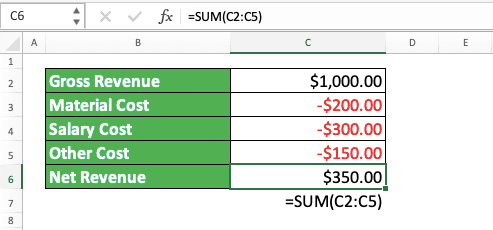Using SUM as a Subtraction Formula in Excel - Compute Expert

# Using SUM as a Subtraction Formula in Excel

Home >> Excel Tutorials from Compute Expert >> Excel Formulas List >> Using SUM as a Subtraction Formula in Excel

In this tutorial, you will learn how to use SUM as a subtraction formula in excel.

When we work with numbers in excel, we sometimes need to subtract them so we can get the result we want. For this subtraction process, we may want to use a built-in excel formula so we can do it faster.

Unfortunately, there isn’t a formula that excel provides to subtract our numbers. However, we have an alternative formula that we can use for the subtraction purpose, which is SUM.

Want to know how to use SUM as a subtraction formula in excel? Read this tutorial until its last part!

Disclaimer: This post may contain affiliate links from which we earn commission from qualifying purchases/actions at no additional cost for you. Learn more

## SUM as a Subtraction Formula?

We use SUM as a subtraction formula? Isn’t SUM the formula we use to sum our numbers in excel?

The primary use of SUM is indeed to sum numbers. However, we can also use it to subtract our numbers in excel.

To do that, we must place a minus symbol ( - ) in the subtractor numbers in our subtraction process. We can place it when we input those numbers into our SUM or in the cells where they are. That way, SUM will sum them as negative numbers, which means they will subtract our positive numbers in our sum process!

## The Way to Write It and Its Inputs

The way to write SUM as a subtraction formula is the same as the way we write it to sum our numbers.

= SUM ( number1 , number2 , … )

However, don’t forget to add a minus symbol to the numbers we use as the subtractors. By doing that, we can do our subtraction process smoothly using SUM.

## Example of Its Usage and Result

The following will give and explain the example of using SUM as a subtraction formula in excel.In this example, you can see how SUM can run our subtraction process.

As we discussed previously, we just need to input all numbers for our subtraction calculation into SUM. Don’t forget to add a minus symbol in front of all the subtractors so SUM can do its job correctly.

## Writing Steps

After we have discussed the SUM writing form, inputs, and implementation example, now let’s discuss its writing steps. If you have used SUM to sum your numbers in excel previously, you should be able to master these steps easily!

1. Type an equal sign ( = ) in the cell where you want to put your subtraction result2. Type SUM (can be with large and small letters) and an open bracket sign after =3. Input the numbers or cell coordinates or cell ranges where you put your numbers. Type comma signs ( , ) between all your inputs.

Don’t forget the minus symbol on the numbers that become the subtractors. You can add the symbol directly in the SUM formula or the numbers’ cell4. Type a close bracket sign after you have inputted all your numbers5. Press Enter
6. Done!## Alternative: Manual Subtraction Formula Writing in Excel

SUM is great for your subtraction process in excel if you have your numbers in a large cell range. You can just input that cell range to SUM without having to input them one by one in your formula writing.

If you involve few numbers in your subtraction process, however, it might be better to type a manual subtraction formula. The manual subtraction formula you type should be something like this.

= number_to_subtract - subtractor

Just don’t forget to type a minus symbol in front of your subtractor if it doesn’t have it yet.

Here is the implementation example of the manual subtraction formula writing in excel.Just use the one which is simpler for your subtraction condition, either SUM or the manual subtraction formula writing!

## Exercise

After you have understood how to use SUM as a subtraction formula, now is the time to do an exercise. You should do this exercise so your understanding of the tutorial lessons can become deeper.

### Questions

Answer the questions in each row in the gray-colored cells using SUM!
1. What is the subtraction calculation result of the 5 numbers in row no. 1? In this row, we have given a minus symbol to all the subtractors
2. What is the subtraction calculation result of the 5 numbers in row no. 2? The subtractors are the second and fifth numbers. In this row, we haven’t given a minus symbol to all the subtractors
3. What is the result if we subtract the no. 2 answer with the no. 1 answer?

Using SUM to subtract your numbers in excel might be easier for financial numbers. We usually already give a minus symbol for expenditures in their cells. Thus, there is no need to give minus symbols anymore just for our SUM writing.

Related tutorials you should learn:

Get updated excel info from Compute Expert by registering your email. It's free!